Page top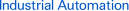# Digital Panel IndicatorsIntroductionFeatures Principles Classifications Engineering DataFurther Information Explanation of Terms Troubleshooting
﻿

RS-232C (Recommended Standard 232C)

RS-232C is a modem interface standard for serial communications defined by the Electronic Industries Alliance (EIA). It defines the electrical specifications, type, and function of the signal line, as well as the mechanical characteristics.

RS-422 and RS-485 (Recommended Standard 422 and 485)

Both RS-422 and RS-485 are standards that specify the electrical characteristics of a balanced differential interface between drivers and receivers defined by the EIA, both are similar in many aspects.
RS-422 allows multiple signal receivers to connect to one driver (signal sender) on the same bus. It does not consider multiple drivers. RS-485 is an extension to RS-422, permitting multiple drivers with tri-state output, and allowing for a multidrop (party line) structure.
It is possible to transmit at a higher speed with an RS-485 compared to the RS-232C standard, which is suitable only for transmission below 20 kbits/s.

The effect from external electromagnetic fields. A type of EMI (Electromagnetic Interference).

Isolation

DC isolation of the input and output signals of a device.
For example, when using a thermocouple to measure the temperature within an electric oven, isolation is used to obtain accurate measurements.

Analog Signal

A signal with a continuous amplitude.

A process monitoring system whereby indicators are installed on the panel and control console to represent different stages of the process. If an error occurs, the corresponding indicator lights and an alarm sounds to provide notification of the error.

EMI (Electromagnetic Interference)

The effect of external electromagnetic fields on device circuits and parts.

Impedance

Refer to Output Impedance and Input Impedance.

SSR (Solid State Relay)

Also called a non-contact relay, a solid state relay is an electronic switch that works without any moving parts. The most common is a photo-triac.

Response

Refer to Frequency Response and Step Response.

Response Time

For a step response, the response time is the time taken for a target value, display value, or an output signal to settle within a specified range of the final value.
(For DC output devices, it often means the time taken for the signal get from 0% to 90%.)

Temperature Coefficient

For the ambient operating temperature of a device, the amount of temperature change due to the ambient temperature deviating from the reference temperature causes changes in the physical properties of the device. The temperature coefficient is the relative change of a physical property when the temperature is changed. (Often indicated as a percentage of the span per unit of temperature.)

Cascade control is a feedback control system that uses the output of one controller to manipulate the set point of other controllers.

When using an OMRON signal generator and measurement device to take measurements under normal operating conditions, accuracy is defined as the difference between the ideal output and the actual output expressed as a percentage of the output span.

The range of load resistance values for which performance is given.

Common Mode Rejection Ratio

Describes how well an instrument can reject the effect of common-mode voltage entering on the input from the output. It is usually expressed in decibels (dB). It is the ratio between the common-mode voltage on the input terminals of the device and the differential input signals required to achieve the same characteristics in the output signal.

Noise voltage caused by external induction appears at the two input terminals. It has the same amplitude and phase at both input terminals. The common-mode voltage is the algebraic average of the instantaneous values of the two voltages.

Error

The difference between measured value, set value, or rated value, and the measured or supplied true value.

Repeatability/Reproducibility

The extent to which the measurements of the same item under the same conditions match when any or all of the following are changed; the person who is taking the measurements, the measuring device, the location, or time. (The degree of repeatability is usually expressed as a percentage of the span.)

The difference between two input terminals when a commonmode voltage is applied to both terminals.

Cyclic Redundancy Check (CRC)

A type of block check for data transmission. It is a popular error checking method as it is simple to implement and has an excellent error detecting ability.

Root-Mean-Square Value

The square root of the mean of the squares of the instantaneous values of AC current or voltage. Also called RMS value.

For a first-order linear time-invariant system, the time constant is the time taken for the step response to reach about 63% of its final value.

Frequency Response

The change in gain and phase of the steady-state output as a response to the input frequency of a sinusoidal wave.

Output Impedance

Impedance of an active device seen from its output terminals.
Like input impedance, it can also be called output resistance.

Output Bias

Output value when the product is idle (i.e., when the input is at the minimum value or there is no input).
For example, if the output is 1 to 5 V, 1 V is the output bias. If the output is 0 to 5 V, 0 V is the output bias.

Signal

Refer to Analog Signal and Digital Signal.

Response of a system to an instantaneous change in input from one constant value to another.

Span

Difference between the maximum and minimum values of a range.
For example, if the range is −15 to 100°C, the span is 115°C.

Split Control

Controlling two or more different elements with one control signal.
For example, for a system that controls hot water temperature with separate control valves for hot and cold water, if both valve position motors are set at 0% to 50%, the hot water valve is controlled open at 100% to 0% but the cold water valve remains at 0%. If the setting is at 50% to 100%, the hot water valve remains at 0% and the cold water valve is controlled open at 0% to 100%.

Control

Refer to Cascade Control, Split Control, and PID Control.

Insulation Resistance

The electrical resistance between two conductors separated by insulating material. The electrical resistance between inputs, outputs, and power source circuits is often of concern for electrical measurements.

Zero Elevation

Shifting the measurement range to the positive direction is called zero elevation.
For example, if the measurement range is −25 to +100°C, zero elevation is 25°C.

Zero Suppression

Shifting the measurement range to the negative direction is called zero suppression.
For example, if the measurement range is 0.2 to 1.0 kgf/cm2, the zero suppression is 0.2 kgf/cm2.

Zero Bias

Zero-suppression and zero-elevation together is called zero bias. (Generally it means that the bias is zero.)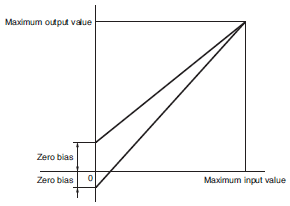Resistance Temperature Sensor

A temperature sensor that uses a resistor element which varies in resistance depending on the temperature. The resistor element may be made from platinum, nickel, or bronze. The platinum type is common used for measurements in the temperature range between −200 and 650°C. In addition to the two-wire configuration, there are three-wire and four-wire configurations to compensate the lead-wire resistances. The three-wire configuration has one line connected to one end of the resistor and two on the other, and the four-wire configuration has two lines connected on either terminals of the resistor.

A technique used to run two or more processes concurrently with one processor by alternating the run time.

Dielectric Strength/Withstand Voltage

The amount of voltage the insulation of an electrical device can withstand in a fixed period of time.

The area between the two set points of a three-position switch.

Linearity

The degree of deviation from a linear relationship between input and output signals. (The degree of linearity is generally indicated as a percentage of the span.)

Signals that express numbers in a discrete state.

Electric Power

The amount of work done by electricity in one unit of time. In other words, the amount of electrical energy consumed in one unit of time.
Refer to Reactive Power, Apparent Power, and Active Power.

Input

Refer to Differential Input and Floating Input.

Input Impedance

Impedance of an active device seen from its input terminals. Often indicated by the equivalent impedance of the parallel resistance and capacitance. For DC measuring devices it is simply called input resistance.

Thermocouple

A thermocouple is a type of temperature sensor that uses two conductors of different metals that generate a voltage across its junction due to the thermoelectric effect. The potential difference across the junction corresponds to the temperature at the measuring junction (thermocouple junction) compared to the temperature at the reference junction (also known as the cold junction), which is held at a constant temperature (e.g., 0°C). The potential difference depends on the type of metals used in addition to the difference in temperatures at the junctions. Common types of thermocouples are R (platinum/platinum rhodium), K (chromel/alumel), E (chromel/constantan), and T (copper/constantan).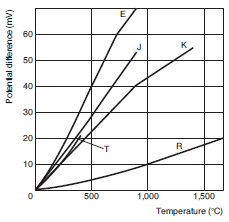Normal Mode Rejection Ratio

Describes how well an instrument can reject the effect of normal-mode voltage entering on the input from the output. It is usually expressed in decibels (dB). It is the ratio between the normal-mode voltage on the input terminals of the device and the increase required in the input signals to achieve the same characteristics in the output signal.

Undesirable input voltage superimposed on the measurement voltage, such as potential difference of the measuring conductors or induction voltage. Also called series mode voltage.

Burnout (Protection)

When there is no input, the output is increased or decreased, to whichever way is safe.
For example, when temperature is controlled using a thermocouple as the sensor, if the thermocouple breaks down due to a burnout, the input is cut off. When this is detected, it may be incorrectly determined as a temperature drop, resulting in the heat controller increasing the temperature and causing overheating. By implementing a burnout protection function, this kind of overheating can be prevented.

Byte

A group of adjacent bits treated as one unit. Often consists of 8 bits.

Bus

A signal communications line where many devices share the same connection. Data can be transferred from any of the signal sources to any of the receivers connected to the bus.

GP-IB: One of the buses established by IEEE-USA. IEEE-488
VME Bus: One of the buses established by IEEE-USA. IEEE-1014
Multibus: One of the buses established by IEEE-USA. IEEE-796

Parity Check

A parity bit is added to a data set as a binary digit to indicate whether the number of ones in a given set of bits is even or odd. It acts as an error detecting code.

A control loop that uses signals proportional to the linear combination of the input, the time integral of the input, and the time derivative of the input to control the output.

Binary Coded Decimal (BCD)

Each digit of a decimal number is represented by four binary bits.
For example, decimal number 23 would be expressed as 0010 0011.

Hysteresis

Properties of equipment and devices where the output value depends on the immediately preceding history of the applied input.

Apparent Power

Apparent power is the simple product of voltage and current supplied to an AC device and is expressed in VA (volt-amperes). It describes the ability of AC devices and power sources to supply current at a given voltage to transformers and motors.

Bit

Short for "binary digit." It is either 1 or 0, and refers to a digit in a binary numeral system. It is the smallest unit of information.

Proportional Band

The range of change in the input (%) required for the output to go from 0% to 100% during proportional action.

The range of input variations where the no change is detected in the output variable. This characteristic is also called the neutral zone.

Frame

In a multiplex structure, a message is transmitted using a time-sharing method. Under this arrangement, a frame is a set of consecutive pulse signals conveying the information on the transmission line.

Floating Input

Input terminals that are isolated from the outer casing, power source, and various output terminals (JIS definition).

There are two ways to assign high and low voltage levels and to the information bits 0 and 1. One is to make 0 correspond to low, and 1 to high, which is called positive logic. The other is in reverse, where 0 corresponds to high and 1 to low, which is called negative logic.

An insulated pair of conductors with similar properties to the thermocouple is connected between the thermocouple terminals and the reference junction to compensate for measurement errors caused by temperature change at the thermocouple terminals.

Reactive Power

The portion of power supply (apparent power) that is actually used by an AC machine is the active power, and the portion of power due to stored energy, which returns to the source in each cycle, is known as reactive power. The unit for reactive power is Var.
It is the product of the voltage and current flowing in the device multiplied by the sine value of the phase difference (θ).
Reactive power Q = Voltage E x Current I x Reactive ratio sinθ (Var)
and
Active power P2 + Reactive power Q2 = Apparent power S2

Active Power

The portion of the power supply that is used by an AC machine is called active power, in units of W (watts). It is the product of voltage, current, and the cosine value of the phase difference (θ). The value cosθ is referred as the power ratio, meaning the portion of power that is useful.

Power Factor

When AC voltage E is applied to a load (the device), the phase of the AC current I flowing in it generally lags behind the voltage E by amount θ. More specifically, when the load is purely resistive, there is no phase shift. When the load is inductive (i.e. a coil), it lags by θ. When the load is capacitive (i.e. a condenser), it leads by θ.

Linearizer

For example with a thermocouple, a detection signal (mV) which has a non-linear relationship with the measurement (temperature) can be used as an input. A linearizer takes this signal and converts it into an output signal that is proportional (linear relationship) to the measured value.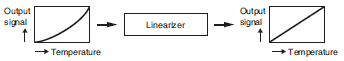Relay Contact

Make contact(normally open (NO) contact)
Break contact(normally closed (NC) contact)
Transfer contact(double-throw contact)

Made from two contacts, one normally open contact and one normally closed contact with a common terminal.

Also called reference junction compensation. When measuring temperature using thermocouples, the reference terminal may not be held at 0°C, but at the surrounding temperature of T1°C instead. Without any compensation, the thermocouple output will be reduced by T1°C. This is compensated by adding potential difference to the internal amplifier corresponding to T1°C.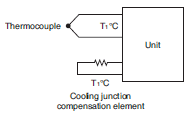Range

The difference between minimum and maximum values that an input or output can reach.

A load cell is a sensor that detects load or force. A strain gauge is a commonly used type of load cell.

Bridge Resistance

The standard resistance seen from the load cell input/output terminals (AB/CD) at ambient temperature. Normally 350 Ω.

Excitation Voltage

Supply voltage applied across the load cell bridge resistance (A?B), normally 5 or 10 V.

Rated Output Voltage

The voltage output when the maximum load corresponding to an additional 1 V is applied to the load cell. Normally 2 mV/V.